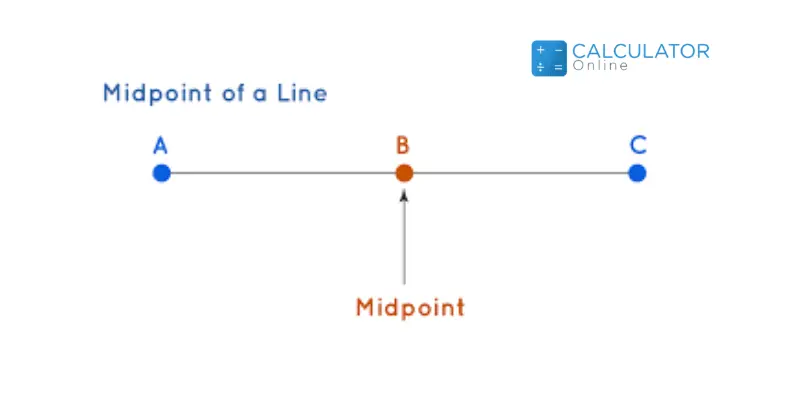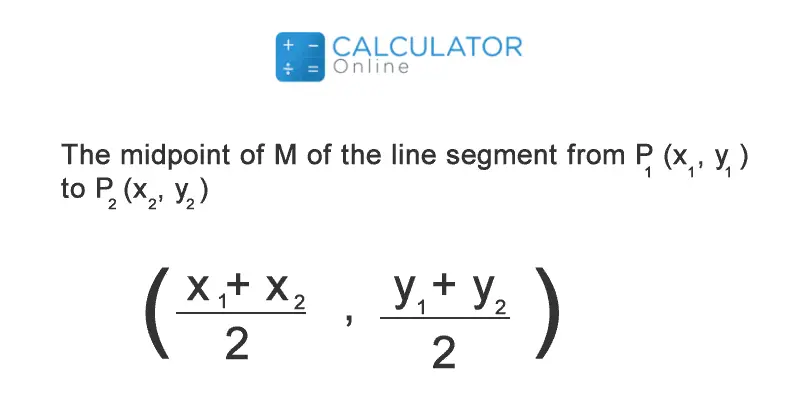•Sign In
• Blog
• Write for UsUh Oh! It seems you’re using an Ad blocker!

We always struggled to serve you with the best online calculations, thus, there's a humble request to either disable the AD blocker or go with premium plans to use the AD-Free version for calculators.

Or# Midpoint Calculator

Provide the coordinates of two points and this midpoint calculator will find coordinates of the midpoint between them up to six dimensions.

Dimensions:

X1

X2

Y1

Y2

Z1

Z2

A1

A2

B1

B2

C1

C2

Table of Content

 1 What Is A Board Foot In Lumber (BF)? 2 Board Foot Formula: 3 Board Foot Units: 4 Important Thickness: 5 How To Calculate Board Feet? 6 What do you mean by the term “Surface Measure”? 7 What is meant by nominal measurement? 8 How do you define lineal measurement?

Get the Widget!

Add this calculator to your site and lets users to perform easy calculations.

Feedback

How easy was it to use our calculator? Did you face any problem, tell us!

Use this free midpoint calculator that helps to find the distance and midpoint of a line segment and shows you the step-by-step calculations.

The midpoint typically useful in geometry and our midpoint coordinate calculator uses the simple midpoint formula geometry to find the missing midpoint coordinates between the points.

So, it’s time to explore the article further to know the terms such as how to find midpoint by using midpoint equation and midpoint calculator, and little much more corresponding to midpoint calculations.

## What is Midpoint?A midpoint exists with any two ordered pairs that lie exactly halfway between each ordered pair. Remember that this is accurate in two dimensions (x and y coordinates) and three dimensions (x, y, and z coordinates). But, don’t fret, since our find the midpoint between two points calculator helps you to find midpoint from 1 to 6 dimensions for the respective coordinates.

When it comes to two dimensions, you only have two endpoints, so remember that the midpoint (or mean) is also referred to as the median, and makes your calculations easy. Midpoints and endpoints are correlated terms, so, try this missing endpoint calculator to find the endpoint of a line segment by using start-point and midpoint coordiantes.

### What is the Midpoint of a Line Segment?In mathematical geometry study, the midpoint is referred to as a point that is halfway between the endpoints in a line segment. More simply, it is said to be the middle point of the line segment.

The midpoint is one that divides the segment into two equal segments. If (x1, y1) and (x2, y2) are the two given endpoints of the line segment, then use the below-mentioned midpoint formula to find the midpoint values for coordinates.

### What is the Midpoint Formula?

The formula for midpoint is taken into account to find the exact center point in a line segment. Use this midpoint equation to calculate the point that bisects a line segment.

Midpoint Formula:

M = (x₁ + x₂/2, y₁ + y₂/2)

Or,

xm = x₁ + x₂/2

ym = y₁ + y₂/2

where;

• M indicated as midpoint
• xm = x coordinate of midpoint
• ym = y coordinate of midpoint

An online midpoint formula calculator also uses the same midpoint formula for finding the midpoint for the given coordinates. So, apart from manual calculations, enter the values into midpoint formula solver to find the midpoints of the line.

You can consider this distance formula calculator online to find the distance among any two points, parallel or straight lines that have coordinates (x, y, z, k) in 1to 4 dimensions.

### How to find the midpoint & Distance with this Midpoint Calculator:

The midpoint solver is 100% free and uses the simple mid point formula to find the exact midpoint between points. Follow the given steps:

Input:

• First of all, you have to choose one dimension (from 1D to 6D) from the drop-down list of this calculator
• Very next, you have to enter the values for the coordinates according to the selected dimension
• Once done, it’s time to hit the calculate button of this calculator

Output:

The distance and midpoints calculator will show:

• Midpoint for the given points
• Step by step calculation by using a formula for the given inputs
• Distance for the given inputs
• Step by step calculation by using distance equation formula
• Also, this midpoint graph calculator shows the graph for the given coordinates

It doesn’t matter whether you want to find midpoint between two points or any points, just select the dimension from the drop-down list add the values and calculate exact midpoint value with this tool.

• This midpoint distance calculator helps to find the distance and midpoint between two points or more
• Work as a midpoint between two numbers calculator to find the distance and midpoint of line segment

## FAQ’s :

### What is the midpoint of 0 and 5?

Only 2.5! To find the midpoint, all you need to add the two numbers together and divide the answer by 2. In this case, 0 + 5 / 2, 5 /2, 2.5.

### What is the midpoint of 30 and 60?

To calculate the midpoint of any two number, all you need to find the average of those two numbers by simply adding them together and dividing the answer by 2. In the given case, 30 + 60 = 90. Also, our midpoint equation calculator uses the simple geometry equation for finding the midpoint between two given coordinates.

### Do you round midpoints?

According to mathematical terms, the boundaries and midpoints should not be rounded off. Remember that these midpoints work out to a value in the hundredths, and even sometimes you need thousandths.

### Is the midpoint The average?

It is very easy to find the midpoint of a line segment by using the x and y coordinates. Finding midpoint becomes easy by taking the average of the x coordinates and simultaneously taking the average of the y coordinates.